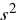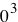# Newton’s Laws Review

1) A ball is held in a person’s hand. A. Identify all the external forces acting on the ball and the reaction to each. B. If the ball is dropped, what force is exerted on it while it is falling? Identify the reaction force for this. (Neglect air resistance)

 A. downward force of gravity reaction force is upward force of ball pulling on Earth Upward force of your hand reaction force is downward force of ball on your handB. downward force of gravity reaction force is upward force of ball pulling on Earth

)

2) In a tug-of-war between 2 athletes, each pulls on the rope with a force of 200 N. What is the tension in the rope? If the rope does not move, what horizontal force does each athlete exert against the ground?

 The tension is the maximum force that occurs in BOTH direction, so the tension is 200 N. If the rope does not move, the net force on each is zero so they must exert 200 N each on the ground.

)

3) A boat moves through the water with 2 forces acting on it. One is a 2000 N forward push by the water on the propeller and the other is an 1800 N resistive force due to the water around the bow.

A. What is the acceleration of the 1000 kg boat?

B. If it starts from rest, how far will it move in 10.0 s?

C. What will its velocity be at the end of this time?

 A. .200 m/B. 10.0 m C. 2.00 m/s

)

4) A 5.0 kg bucket of water is raised from a well by a rope. If the upward acceleration of the bucket is 3.0 m/, find the force exerted by the rope on the bucket.

5) A 40.0 kg wagon is towed up a hill inclined at 18.5° with respect to the horizontal. The tow rope is parallel to the incline and has a tension of 140 N in it. Assume that the wagon starts from rest at the bottom of the hill and neglect friction. How fast is the wagon going after moving 80.0 m up the hill?

6) A 1000 kg car is pulling a 300 kg trailer. Together the car and trailer have an acceleration of 2.15 m/in the forward direction. Neglecting frictional forces on the trailer, determine

A. the net force on the car

B. the net force on the trailer

C. the force exerted by the trailer on the car

D. the resultant force exerted by the car on the road

Answer: (A. 2.15 X 1N forward

B. 645 N forward

C. 645 N backwards

D. 1.02 X 1N at 74.1° above the horizontal rearward)

7) A 72 kg man stands on a spring scale in an elevator. Starting from rest, the elevator ascends, attaining its maximum speed of 1.2 m/s in 0.08 s. It travels with this constant speed for 5.0 s, undergoes a uniform negative acceleration for 1.5 s and comes to rest. What does the spring scale register A. before the elevator starts to move? B. during the first 0.80 s? C. while the elevator is traveling at constant speed? D. during the negative acceleration?

Answer: (A. 7.1 X 1N

B. 1.8 X 1N

C. 7.1 X 1N

D. 6.5 X 1N

)

8) Two people pull as hard as they can on ropes attached to a 200 kg boat. If they pull in the same direction, the boat has an acceleration of 1.52 m/to the right. If they pull in opposite directions, the boat has an acceleration of 0.518 m/to the left. What is the force exerted by each person on the boat? (Disregard any other forces on the boat.)